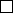3Lagrange's Theorem

IA Groups3.1 Small groups
We will study the structures of certain small groups.
Example
(Using Lagrange theorem to find subgroups)
.
To find subgroups of
D
10
, we know that the subgroups must have size 1, 2, 5 or 10:
1: {e}
2: The groups generated by the 5 reflections of order 2
5:
The group must be cyclic since it has prime order 5. It is then generated
by an element of order 5, i.e.
r, r
2
, r
3
and
r
4
. They generate the same
group hri.
10: D
10
As for D
8
, subgroups must have order 1, 2, 4 or 8.
1: {e}
2: 5 elements of order 2, namely 4 reflections and r
2
.
4:
First consider the subgroup isomorphic to
C
4
, which is
hri
. There are two
other non-cyclic group.
8: D
8
Proposition. Any group of order 4 is either isomorphic to C
4
or C
2
× C
2
.
Proof.
Let
|G|
= 4. By Lagrange theorem, possible element orders are 1 (
e
only),
2 and 4. If there is an element a G of order 4, then G = hai
=
C
4
.
Otherwise all non-identity elements have order 2. Then
G
must be abelian
(For any
a, b
, (
ab
)
2
= 1
ab
= (
ab
)
1
ab
=
b
1
a
1
ab
=
ba
). Pick
2 elements of order 2, say
b, c G
, then
hbi
=
{e, b}
and
hci
=
{e, c}
. So
hbi hci
=
{e}
. As
G
is abelian,
hbi
and
hci
commute. We know that
bc
=
cb
has
order 2 as well, and is the only element of
G
left. So
G
=
hbi × hci
=
C
2
× C
2
by the direct product theorem.
Proposition.
A group of order 6 is either cyclic or dihedral (i.e. is isomorphic
to C
6
or D
6
). (See proof in next section)